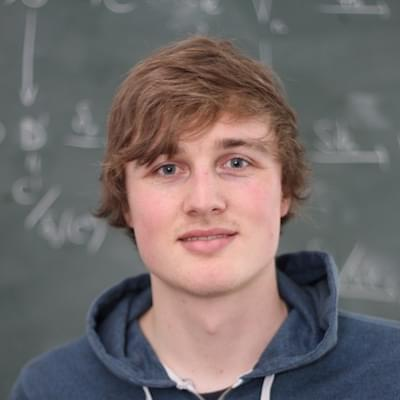# Daniel LütgehetmannI am researching artificial intelligence based on neuroscientific insights at INAIT. This page reflects my previous academic work and is kept online just for reference. In my last academic position I was a postdoc at the Institute of Mathematics at the University of Aberdeen. I did my PhD at Berlin Mathematical School under the supervision of Holger Reich.

My main research interests were configuration spaces of graphs and Teichmüller theory.

Here are the slides of my talk at the Young Topologist Meeting 2017.

Documents
Topological complexity of configuration spaces of fully articulated graphs and banana graphsjoint with David Recio-Mitter
In this paper we determine the topological complexity of configuration spaces of graphs which are not necessarily trees, which is a crucial assumption in previous results. We do this for two very different classes of graphs: fully articulated graphs and banana graphs. We also complete the computation in the case of trees to include configuration spaces with any number of points, extending a proof of Farber. At the end we show that an unordered configuration space on a graph does not always have the same topological complexity as the corresponding ordered configuration space (not even when they are both connected). Surprisingly, in our counterexamples the topological complexity of the unordered configuration space is in fact smaller than for the ordered one. ( link )
Thesis: Representation Stability for Configuration Spaces of Graphs
We consider for two based graphs G and H the sequence of graphs Gk given by the wedge sum of G and k copies of H. We show that the sequence of representations of the symmetric group given by the rational homology of the configuration space of these spaces is representation stable in the sense of Church and Farb. Furthermore, we extend this result to more general stabilization of the graph. We also prove that for 3-vertex connected graphs the sequence of first cohomology groups of configuration spaces of these graphs induced by forgetting particles satisfies representation stability. ( link )
Representation Stability for Configuration Spaces of Graphs
We consider for two based graphs G and H the sequence of graphs Gk given by the wedge sum of G and k copies of H. We show that the sequence of representations of the symmetric group given by the rational homology of the configuration space of these spaces is representation stable in the sense of Church and Farb. ( link )
The Homology of Configuration Spaces of Graphsjoint with Safia Chettih
We show that the homology of configuration spaces of finite graphs is torsion free. Furthermore, we give a concrete generating set for the homology of configuration spaces of finite trees. ( link )
Master's Thesis: Configuration Spaces of Graphs
We investigate the cohomology of configuration spaces of graphs and its behaviour when stabilizing the number of particles. ( pdf )
Bachelor's Thesis: The Lipschitz Distance on Outer Space
We give an overview on the Lipschitz distance on Outer Space. ( pdf )
Media
Cycles of the Homology of Configuration Spaces of Graphs
This is an animation of a basis of the integral homology of the configuration spaces of certain graphs. We calculated the homology with the help of CHomP and Sage.
Travel